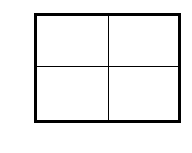### Home > CAAC > Chapter 6 > Lesson 6.2.3 > Problem6-66

6-66.
1. Use generic rectangles to multiply each of the following expressions. Homework Help ✎

1. (x + 2)(x − 5)

2. (y + 2x)(y + 3x)

3. (3y − 8)(−x + y)

4. (x − 3y)(x + 3y)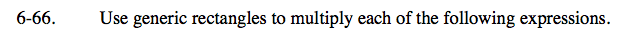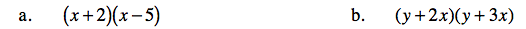Draw a generic rectangle.

Multiply the value of the column and the value of the row to find the value of each box in the rectangle.

(x + 2)(x − 5) = x² − 3x − 10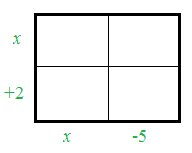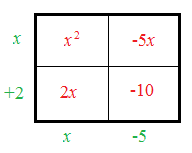See the hints in part (a).

(y + 2x)(y + 3x) = y² + 5xy + 6x²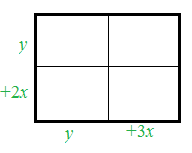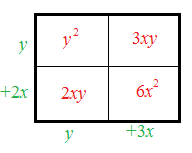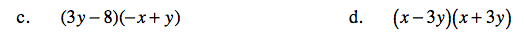See the hints in parts (a) and (b).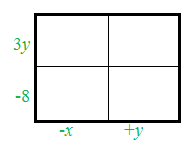See the hints in part (a).